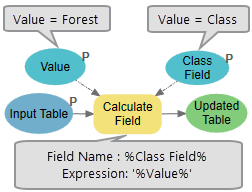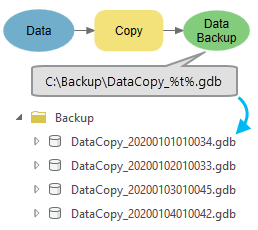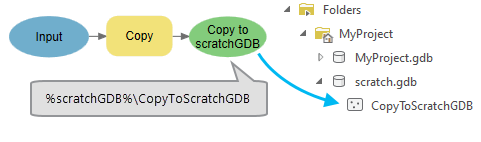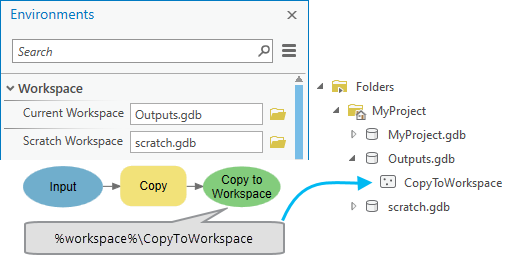# Inline variable substitution

In ModelBuilder, the value or dataset path of a variable can be substituted for another variable by enclosing the substituting variable name in percent signs (%VariableName%). Substituting variables in this manner is called inline variable substitution.

For example, if you have a variable Name with a value of Wilson, you can construct a dataset path as C:\Data\Output.gdb\Clipped_%Name% and the path will be resolved as C:\Data\Output.gdb\Clipped_Wilson.

ModelBuilder supports inline variable substitution in the following formats:

• Model variables—Any variable in a model.
• System variables—Variable %n% can be used in an iteration workflow as an iteration counter in the model. The variable %t% refers to the time when the output is generated in the format of YYYYMMDDHHMMSS.
• Workspace environments— Four workspace environments (%scratchGDB%, %scratchFolder%, %workspace%, and %scratchWorkspace%) can be used in ModelBuilder to simplify model data management.

## Model variable substitution

In the model illustrated below, a workspace variable, Data Workspace, has a value of C:\Data.gdb. This workspace location is substituted as an inline variable in the Project tool parameters by enclosing the variable name in percent symbols. At run time, the actual variable value, C:\Data.gdb, is substituted for %Data Workspace%.

### Use with iterator

The use of model variable substitution is especially important when working with ModelBuilder iterators. When the iterator Iterate Feature Classes runs, it creates an output variable for both the path and name of each feature class in a workspace. The value in the Name variable can be used to construct the path to the output Projected Feature Class. When the tool executes, %Name% is replaced with the name of the feature class.

### User input to a model tool

Model variable substitution can help you pass values entered by a user directly into a tool inside your model. For example, in the model illustrated below, Parcel ID is a model parameter that is specified when the model tool is run from the Geoprocessing pane. This variable is used in the Expression parameter of the Select Layer By Attribute tool as "Parcel" = '%Parcel ID%'. When the tool runs, %Parcel ID% in the expression is replaced with the parcel ID (9 in the case below), and only those parcels with an ID of 9 are selected.

##### Note:
The Parcel ID variable in this example is a string. Inline variables that are strings need to be enclosed within quotation marks ('%string variable%') in an expression. Inline variables that are numbers do not require quotation marks.

In a second example, variable substitution is used to pass values to the parameters of the Calculate Field tool. The model illustrated below contains two variables, Class Field and Value. Class Field with the value of Class is used as the Field Name parameter. The Value variable with the value of Forest is used as the Expression parameter. When the Calculate Field tool runs, the Class field gets the value of Forest that saves in the Value variable.### Use with Calculate Value

Calculate Value is a ModelBuilder utility that allows you to calculate a value based on a Python expression and use that value in your model. You can use model variable substitution to pass values from model variables into the Calculate Value Python expression, or use the output variable name that stores the calculated value in another tool in your model.

The model below contains two numeric variables: Number of Residents and Waste Per Person Per Year. You can use these variables in the Calculate Value tool expression by enclosing them in percent symbols. When the Calculate Value tool runs, the variable names will be substituted with their values and multiplied together to calculate Total Waste Per Year.

### Variable substitution with file extensions

If a variable points to a file with an extension, for example, elevation.tif, and this variable needs to be included in the output path of another tool using inline substitution, the output erroneously includes the .tif extension in the output name. In such cases, the Parse Path tool can be used to get only the file name: elevation. The Parse Path tool's output Name variable can then be used as inline substitution into the next tool.

## System variable substitution

ModelBuilder provides system variables that you can use in a model to represent the current model iteration (%n%) and time (%t%).

The variable %n% refers to the current model iteration (the first iteration is zero) when a model contains an iterator. For example, the For iterator is used to iterate a model four times. The output of the Buffer tool is used as feedback into the tool as input. The model iterates and creates an output at each iteration. %n% is used in the output name of Buffer to give the output of each iteration a new name.

The variable %t% refers to the time when tool output is created. For example, if a model is scheduled to run daily for data backup, using the variable %t% creates an output with a time stamp to indicate the backup date.##### Legacy:

The variable %i% works the same as %n%. It is designed for ArcMap, thus not recommended for use on ArcGIS Pro.

## Workspace environments

You can use four workspace environments in ModelBuilder to simplify data management within a model. You can use workspace environments in model parameters and run models from the Geoprocessing pane.

EnvironmentUsageLocationRecommend usage

Scratch GDB

%scratchGDB%

Project Folder\scratch.gdb

Intermediate outputs

Scratch Folder

%scratchFolder%

Project Folder\scratch

File-based intermediate outputs

Current Workspace

%workspace%

Current Workspace environment setting

Geoprocessing tool inputs and outputs

Scratch Workspace

%scratchWorkspace%

Scratch Workspace environment setting

Intermediate outputs

For example, if a tool output is specified as %scratchGDB%\CopyToScratchGDB, a scratch.gdb geodatabase is created, if it doesn't already exist, and the output CopyToScratchGDB is created in the Scratch GDB environment geodatabase.In the example below, if a tool output is specified as %workspace%\CopyToWorkspace, the output CopyToWorkspace is created in the Current Workspace environment location.